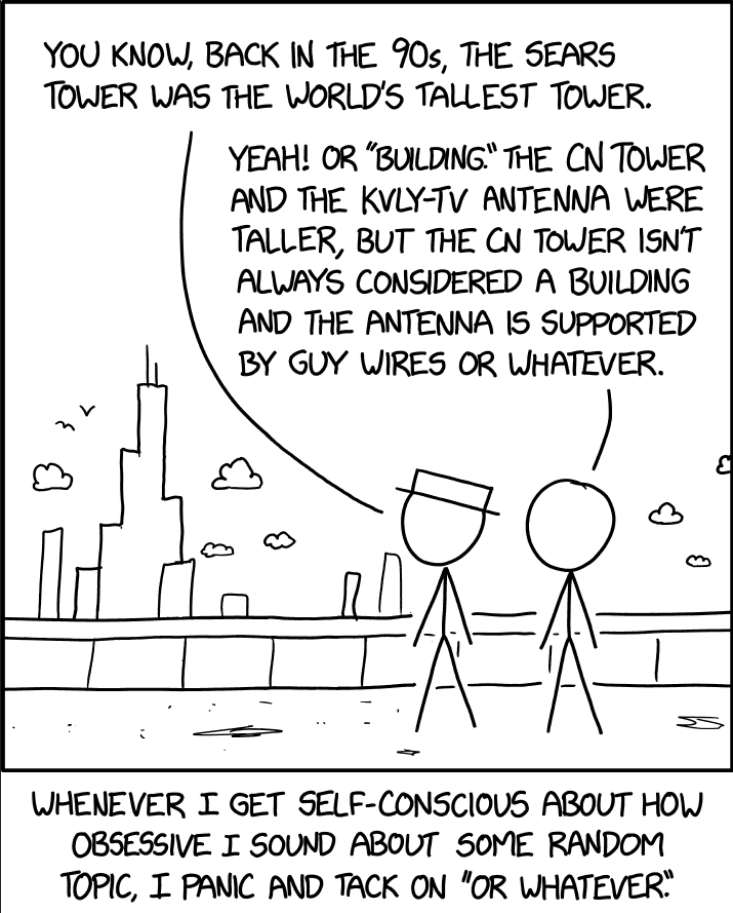# How to Convert a List of Lists to a Set in Python?

## Problem Formulation

💬 Question: Given a list of lists (=nested list) in Python. How to convert this list of lists to a set in Python?

This is not a trivial problem because Python lists are mutable and, therefore, not hashable. And because of that, they cannot be converted to a set using the direct `set()` approach like so:

```my_list = [[1, 2, 3], [4, 5, 6], [7, 8, 9]]
my_set = set(my_list)```

This raises the `TypeError: unhashable type: 'list'` as can be seen in the output when executing the previous code snippet:

```Traceback (most recent call last):
File "...\code.py", line 2, in <module>
my_set = set(my_list)
TypeError: unhashable type: 'list'```

Let’s have a look at different ways to resolve this problem and convert a nested list to a set in Python.

## Method 1: Set Comprehension + tuple()

To convert a list of lists `my_list` to a set in Python, you can use the expression `{tuple(x) for x in my_list}` that goes over all inner lists in a set comprehension and converts each of them to a tuple. Tuples are immutable, hashable, and therefore can be used in sets, unlike lists.

Here’s the code example:

```my_list = [[1, 2, 3], [4, 5, 6], [7, 8, 9]]
my_set = {tuple(x) for x in my_list}
print(my_set)
# {(7, 8, 9), (1, 2, 3), (4, 5, 6)}```

Notice how the order of the elements is lost after converting the list of lists to a set!

## Method 2: Generator Expression + set() + tuple()

A variant of the previous approach is the following:

To convert a nested `my_list` to a set in Python, use the expression `set(tuple(x) for x in my_list)` that iterates over all inner lists in a generator expression passed into the `set()` function and convert each of them to a tuple using `tuple()` built-in function.

Again, tuples are immutable, hashable, and therefore can be used in sets, unlike lists.

```my_list = [[1, 2, 3], [4, 5, 6], [7, 8, 9]]
my_set = set(tuple(x) for x in my_list)
print(my_set)
# {(7, 8, 9), (1, 2, 3), (4, 5, 6)}
```

Super concise one-liner, isn’t it?

## Method 3: Loop + Convert + Add Tuples

A simple and straightforward approach to convert a Python list of lists to a set is in four steps:

1. Initialize an empty set using `set()`,
2. Iterate over the inner list in a simple `for` loop,
3. Convert the inner list to an immutable tuple using `tuple()`, and
4. Add the newly-created tuple to the set using `set.add()`.

Here’s the code example:

```my_list = [[1, 2, 3], [4, 5, 6], [7, 8, 9]]

my_set = set()
for x in my_list:

print(my_set)
# {(7, 8, 9), (1, 2, 3), (4, 5, 6)}
```

🌎 Learn More: You can learn more about the `set.add()` method in this Finxter blog tutorial.

## Method 4: Flatten List of Lists + Set Comprehension

If you need to flatten the nested list before converting it to a flat set, you can use the set comprehension `{i for lst in my_list for i in lst}` with nested one-line `for` loop expression to iterate over all inner elements of the list of lists.

Here’s the code:

```my_list = [[1, 2, 3], [4, 5, 6], [7, 8, 9]]

my_set = {i for lst in my_list for i in lst}

print(my_set)
# {1, 2, 3, 4, 5, 6, 7, 8, 9}
```

Here’s a background video on flattening a list of lists:

## Method 5: Convert Inner Lists to Strings

An alternative approach to convert a list of lists to a set is to convert the inner lists to strings because strings are immutable and hashable and can therefore be used as set elements:

``{str(lst) for lst in my_list}``

We use the `str()` function in a set comprehension statement to convert the list of lists to a set of strings.

Here’s the code example:

```my_list = [[1, 2, 3], [4, 5, 6], [7, 8, 9]]

my_set = {str(lst) for lst in my_list}

print(my_set)
# {'[7, 8, 9]', '[1, 2, 3]', '[4, 5, 6]'}
```

🌎 Learn More: If you want to convert the string representation of the list back to a list, you can find out more in this Finxter tutorial.

## Summary

The five most Pythonic ways to convert a list of lists to a set in Python are:

Feel free to check out more free tutorials and cheat sheets for learning and improving your Python skills in our free email academy:

## Nerd HumorOh yeah, I didn’t even know they renamed it the Willis Tower in 2009, because I know a normal amount about skyscrapers. — xkcd (source)